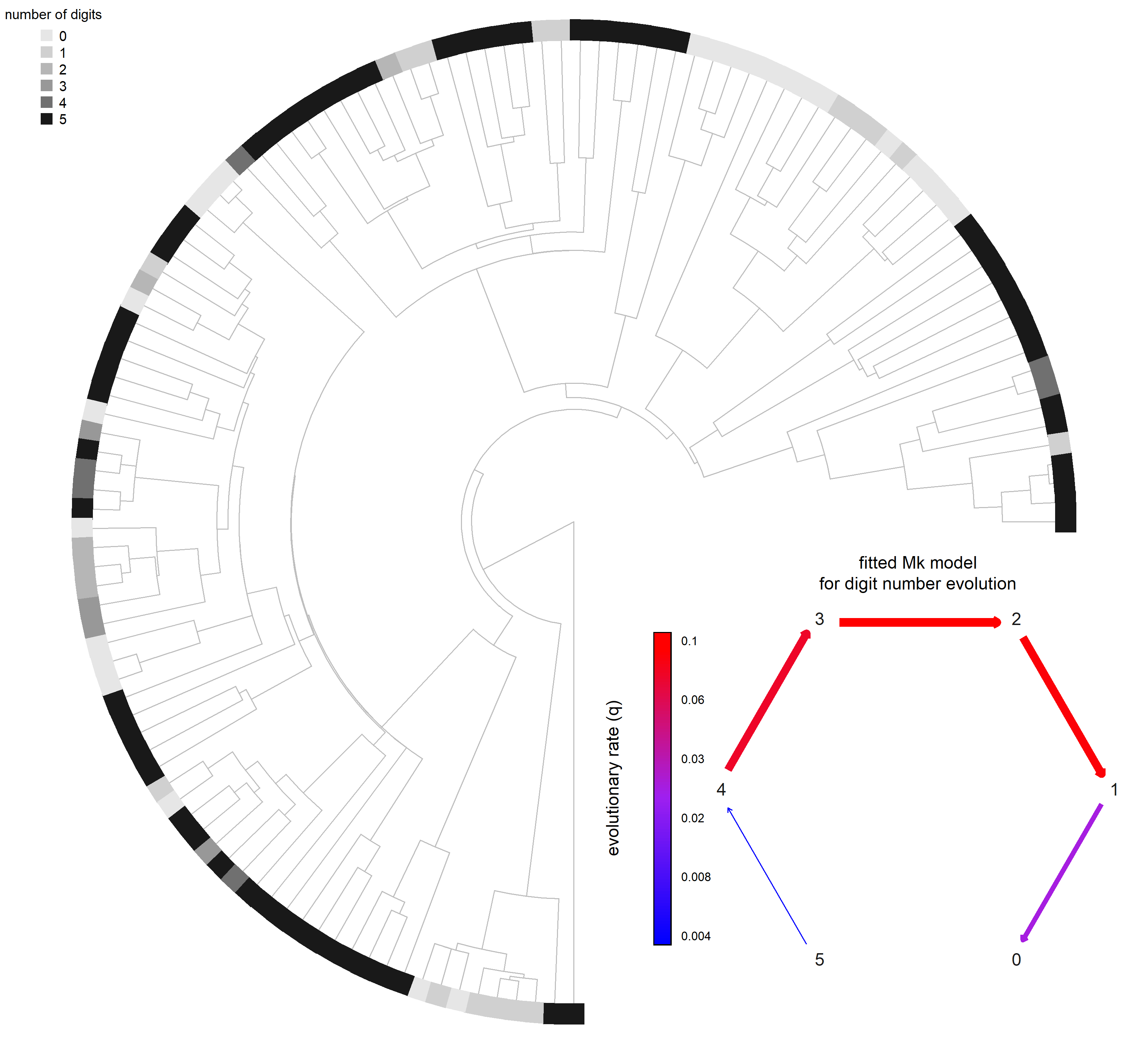## Wednesday, September 14, 2022

### On the expected distribution of the residual error from phylogenetic regression & ANOVA

A phytools user recently asked the following interesting question:

“I contact you for a statistical question regarding GLS and PGLS, which I am not super familiar with. I have read both that GLS do and do not require the distribution of residuals to be normal, and I cannot make a decision on this. A reviewer even ask us to test for normality of the variables, not even the residuals. What is your opinion on this?”

As I have both posted on this blog and published about in the past:

“…we do not expect normality of the dependent (or independent, for continuous x) variables in phylogenetic data. Instead, what we do expect is multivariate normality of the residual error in y given X (or, equivalently, normality of y given X, controlling for the tree).”

If we want to test for normality, we should test for the multivariate normality of the residual errors of our fitted model. One way to do that is to back-transform our data using a decomposition (like a Cholesky factorization) of the phylogenetic covariance matrix (e.g., following Butler et al 2000), and then test for normality of these transformed residuals. This is functionality (& philosophically) equivalent to a simpler normalization of uncorrelated random data.

Here's a quick example, using simulation. In this case, I'll use an independent variable, X, that is a factor and then do a phylogenetic generalized ANOVA, but the same analysis would apply with a GLS regression. For my normality test, I will use the function `lillie.test` of the nortest R package on CRAN.

``````## load packages
library(phytools)
library(nlme)
library(nortest)
## simulate tree
tree<-NULL
while(is.null(tree))
tree<-pbtree(b=1,d=0.8,t=20,
extant.only=TRUE)
``````
``````## Warning:
##   no extant tips, tree returned as NULL
``````
``````## simulate phylogenetic residual error
e<-fastBM(tree,sig2=2)
## simulate factor
X<-setNames(as.factor(sample(1:3,Ntip(tree),
replace=TRUE)),tree\$tip.label)
## arbitrary beta
beta<-c(5,-10,30)
## simulate y
y<-beta[X]+e
## put data in data frame
Data<-data.frame(x=X,y=y)
## create correlation structure
spp<-rownames(Data)
bm<-corBrownian(1,tree,form=~spp)
## fit generalized anova using nlme::gls
fit.anova<-gls(y~x,data=Data,correlation=bm)
fit.anova
``````
``````## Generalized least squares fit by REML
##   Model: y ~ x
##   Data: Data
##   Log-restricted-likelihood: -661.9959
##
## Coefficients:
## (Intercept)          x2          x3
##    7.775999  -14.964163   24.984617
##
## Correlation Structure: corBrownian
##  Formula: ~spp
##  Parameter estimate(s):
## numeric(0)
## Degrees of freedom: 355 total; 352 residual
## Residual standard error: 5.418697
``````

Now that I've fit my model, I'm going to visualize and then test for normality of three different vectors. First, the original data for my dependent variable, y; second, my untransformed residuals from the fitted model; last, my transformed residuals in which I use the Cholesky factorization of the inverse of my phylogenetic covariance matrix, `vcv(tree)`.

``````## original response variable, y
d1<-density(y,bw=1)
plot(d1,bty="n",type="n",main="density of y",
font.main=3)
polygon(d1,col=make.transparent("blue",0.25),
border="blue")
``````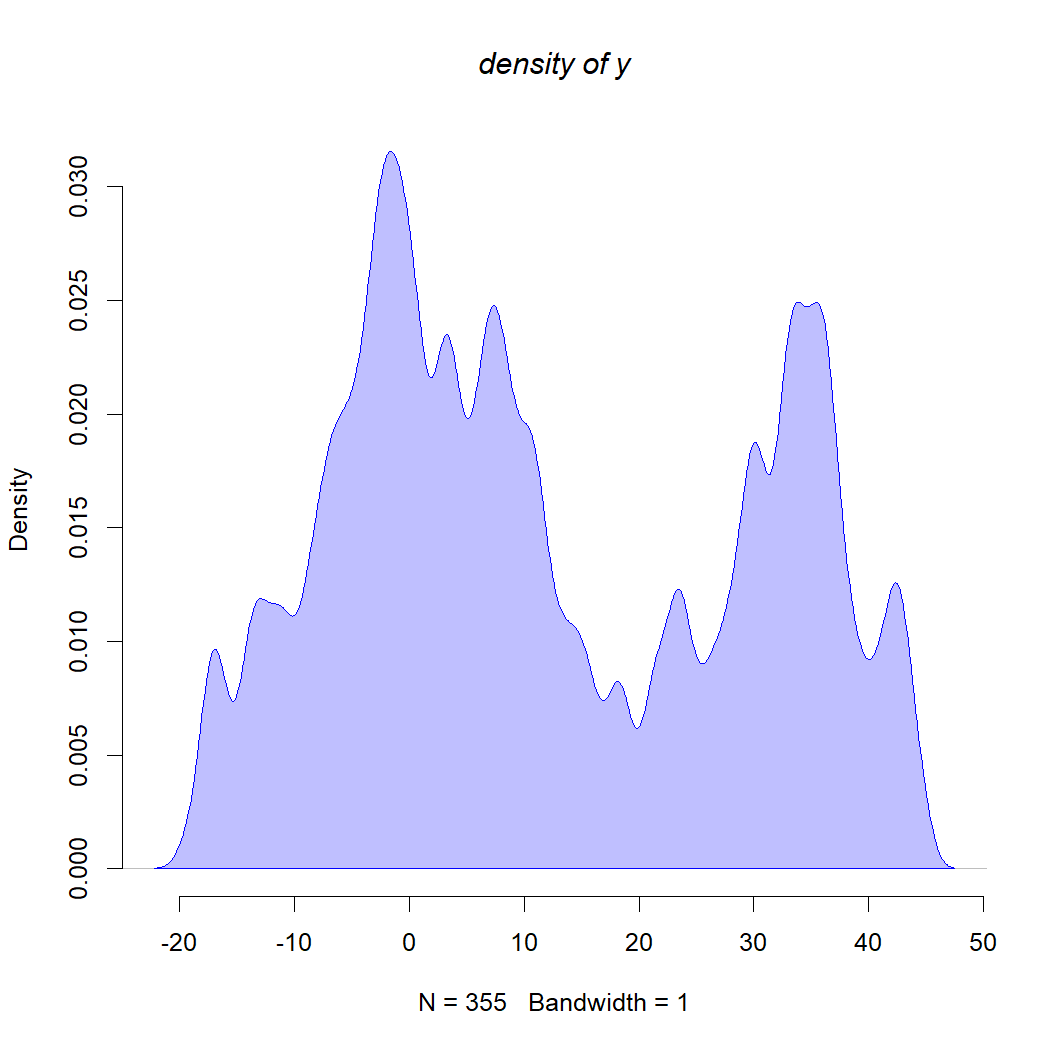``````## normality test (always fails)
lillie.test(y)
``````
``````##
##  Lilliefors (Kolmogorov-Smirnov) normality test
##
## data:  y
## D = 0.099288, p-value = 5.66e-09
``````
``````## untransformed residuals
d2<-density(residuals(fit.anova),bw=1)
plot(d2,bty="n",type="n",main="density of untransformed residuals",
font.main=3)
polygon(d2,col=make.transparent("blue",0.25),
border="blue")
``````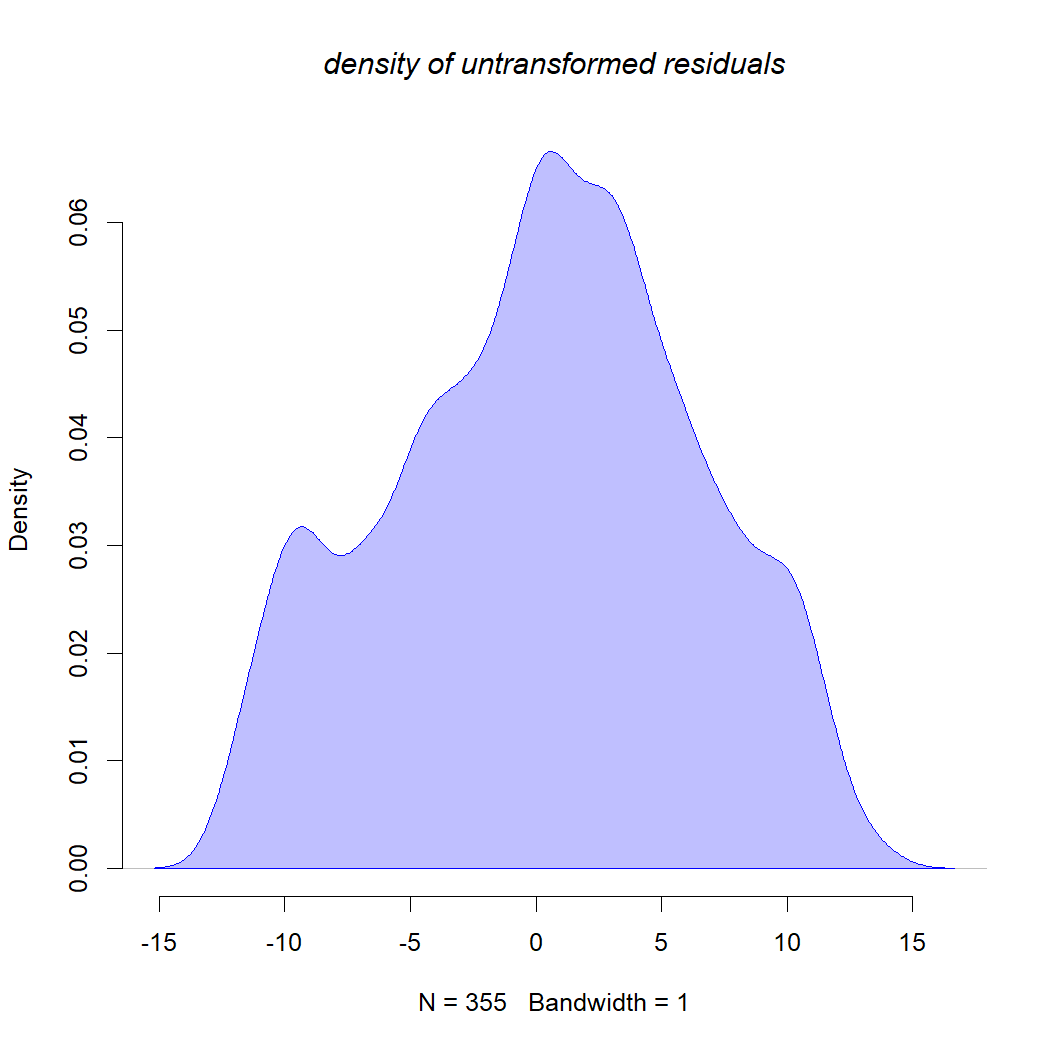``````## normality test (often fails)
lillie.test(residuals(fit.anova))
``````
``````##
##  Lilliefors (Kolmogorov-Smirnov) normality test
##
## data:  residuals(fit.anova)
## D = 0.040746, p-value = 0.162
``````
``````## transformed residuals
transformed<-chol(solve(vcv(tree)))%*%residuals(fit.anova)
d3<-density(transformed,bw=1)
plot(d3,bty="n",type="n",main="density of transformed residuals",
font.main=3)
polygon(d3,col=make.transparent("blue",0.25),
border="blue")
``````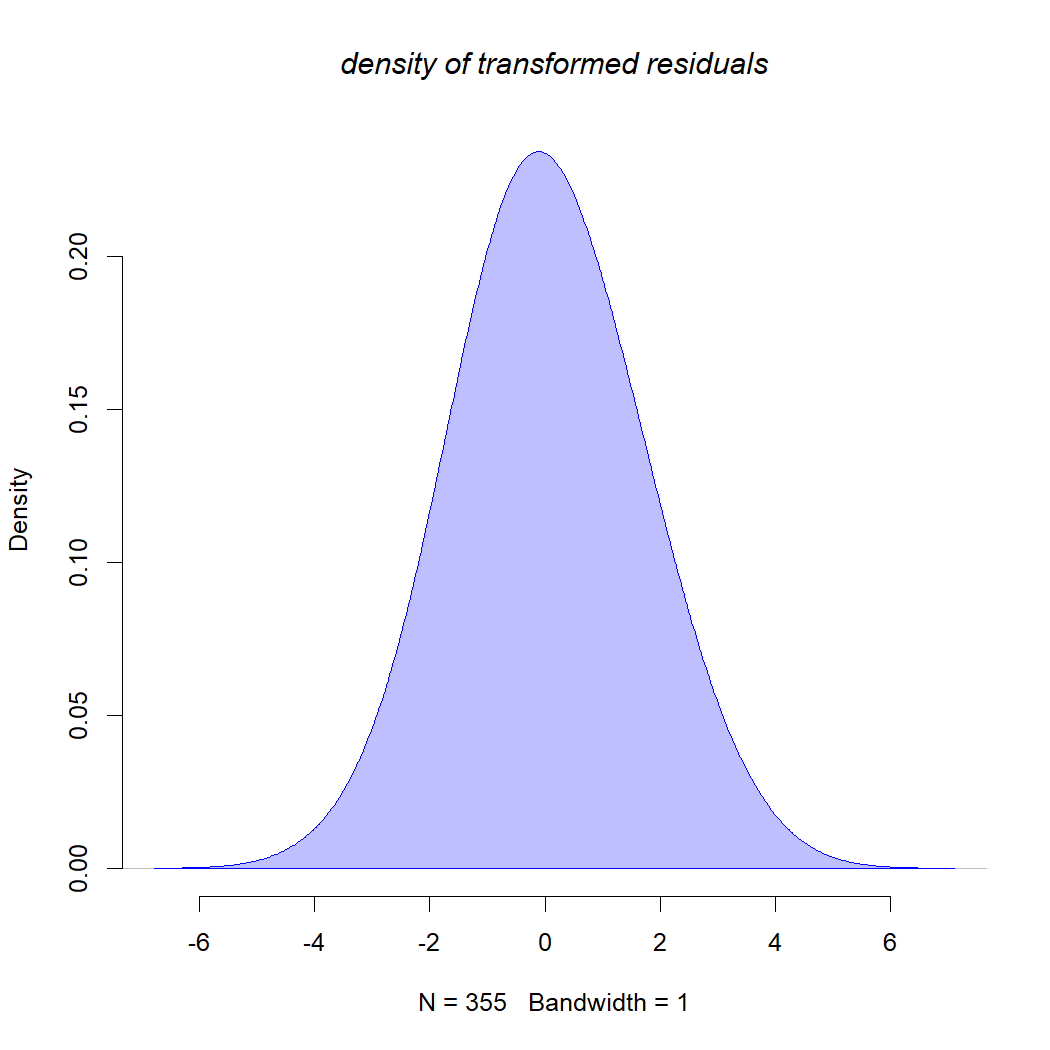``````## normality test (usually passes)
lillie.test(transformed)
``````
``````##
##  Lilliefors (Kolmogorov-Smirnov) normality test
##
## data:  transformed
## D = 0.041221, p-value = 0.1506
``````

OK, now let's try a “real” example, in this case from my book with Luke Harmon using data from Kirk & Kay (2004).

``````## load data
row.names=1,stringsAsFactors=TRUE)
## create correlation structure
spp<-rownames(primate.data)
corBM<-corBrownian(phy=primate.tree,form=~spp)
## fit model
primate.ancova<-gls(log(Orbit_area)~log(Skull_length)+
Activity_pattern,data=primate.data,
correlation=corBM)
summary(primate.ancova)
``````
``````## Generalized least squares fit by REML
##   Model: log(Orbit_area) ~ log(Skull_length) + Activity_pattern
##   Data: primate.data
##         AIC       BIC   logLik
##   -86.45385 -74.18212 48.22693
##
## Correlation Structure: corBrownian
##  Formula: ~spp
##  Parameter estimate(s):
## numeric(0)
##
## Coefficients:
##                                Value Std.Error   t-value p-value
## (Intercept)               -0.7310348 0.3761724 -1.943350  0.0552
## log(Skull_length)          1.4717247 0.0758534 19.402228  0.0000
## Activity_patternDiurnal   -0.1060187 0.0777268 -1.363991  0.1761
## Activity_patternNocturnal  0.2935941 0.1213931  2.418540  0.0177
##
##  Correlation:
##                           (Intr) lg(S_) Actv_D
## log(Skull_length)         -0.914
## Activity_patternDiurnal   -0.277  0.100
## Activity_patternNocturnal -0.518  0.329  0.602
##
## Standardized residuals:
##         Min          Q1         Med          Q3         Max
## -1.89188666 -0.77574126 -0.41922692  0.07417665  2.26411750
##
## Residual standard error: 0.2933082
## Degrees of freedom: 90 total; 86 residual
``````
``````## test for normality of transformed residuals
transformed<-chol(solve(vcv(primate.tree)))%*%residuals(primate.ancova)
d3<-density(transformed,bw=0.05)
plot(d3,bty="n",type="n",main="density of transformed residuals (primate ANCOVA)",
font.main=3)
polygon(d3,col=make.transparent("blue",0.25),
border="blue")
``````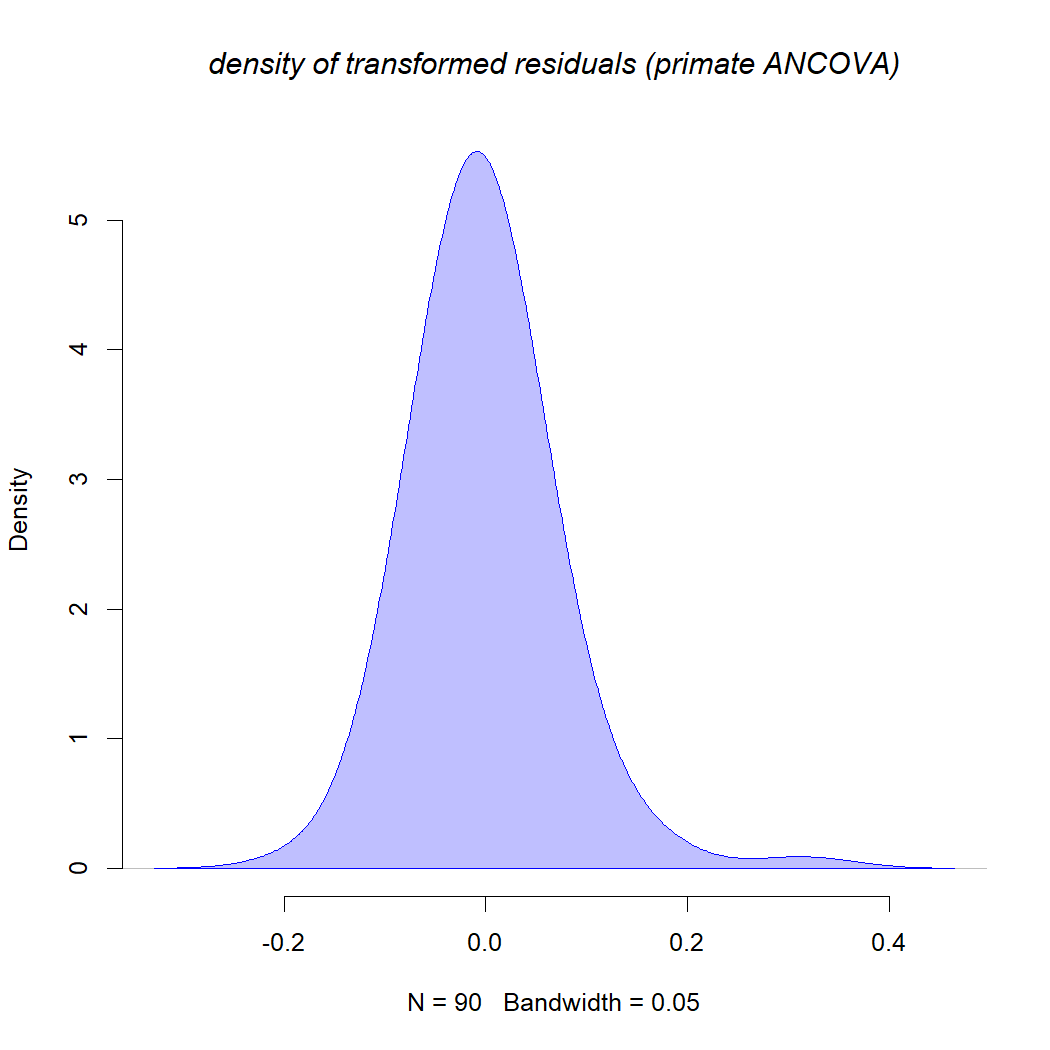``````lillie.test(transformed)
``````
``````##
##  Lilliefors (Kolmogorov-Smirnov) normality test
##
## data:  transformed
## D = 0.094818, p-value = 0.04442
``````

So, in this case we see that our transformed residuals fail normality, although the failure is not too severe so (just as with OLS) we should not be overly concerned, IMO.

That's it!

## Thursday, September 8, 2022

### Updated default orientation of plotted Q matrix, for discrete character evolution (Mk models) -- and a new option to add them to an existing plot!

I recently learned on Twitter of a new paper by Kate Thomas et al. (2022) modeling the evolution of pupil shape and orientation across amphibians.

One thing that jumped out at me straight away, and reminded me of something that I've been meaning to do for a long time, was the orientation of their graphed Q matrix (and its superposition on top of the plotted tree).

Even though I have recently added a bunch of new cool functionality to the `plot.Qmatrix` method in phytools (e.g., 1, 2, 3), the default orientation of the plotted Q matrix (that is, how it is rotated around the origin) is fixed.

Since the default orientation is to start at 0 and then evenly space the trait space in an even-angle fashion, especially for low dimensional (e.g., 3 × 3) Q matrices, this can create a weird visual impression that the plotted matrix is somehow 'off-kilter'.

For example, take a look at this plotted 3 × 3 matrix from an earlier post on this blog.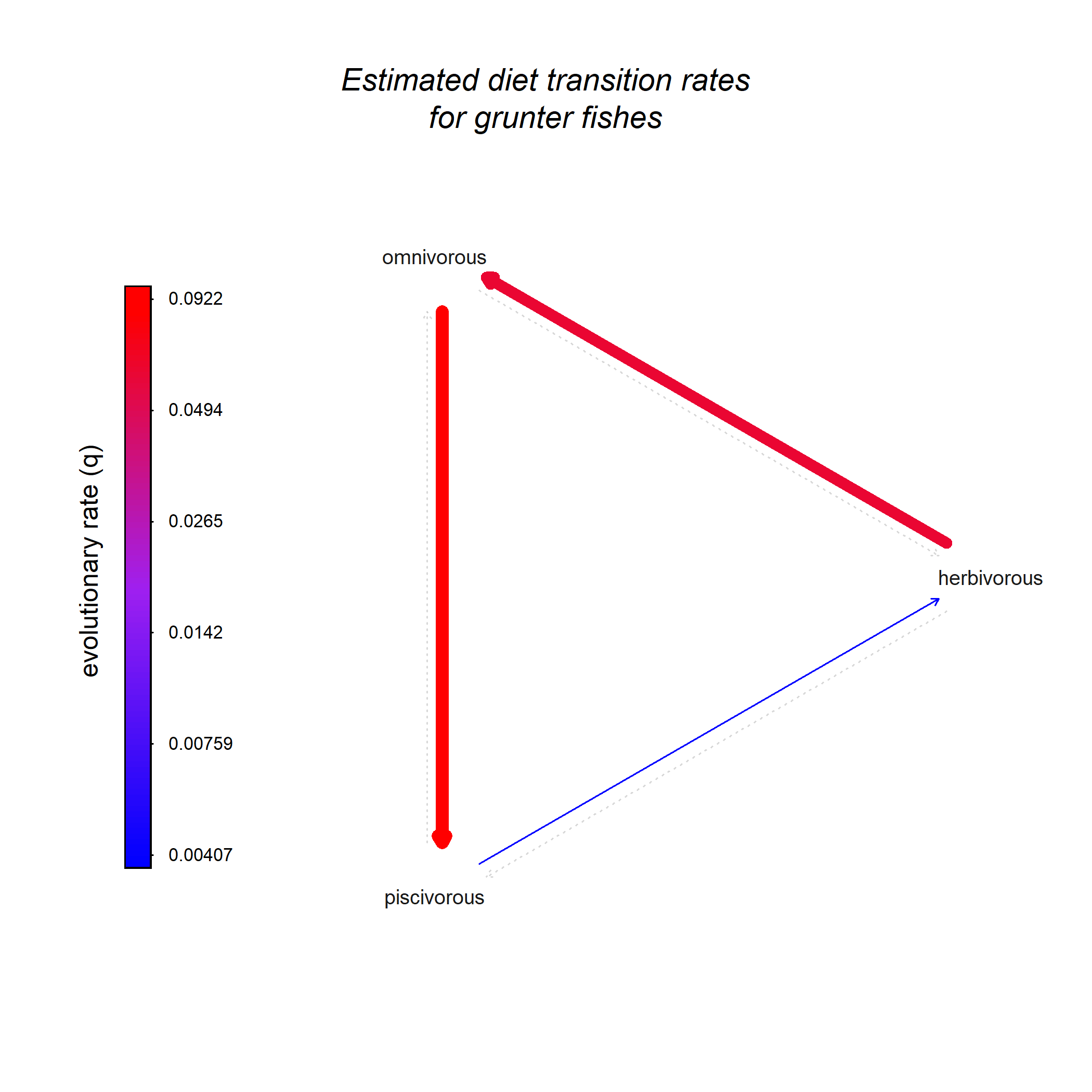Here's what the update looks like – using the argument `rotate=0` to show the “old” plot orientation as well as the new default.

``````library(phytools)
``````
``````## Loading required package: ape
``````
``````## Loading required package: maps
``````
``````packageVersion("phytools")
``````
``````##  '1.2.3'
``````
``````Q2<-as.Qmatrix(matrix(c(
-0.9,0.9,
1.2,-1.2),2,2,byrow=TRUE,
dimnames=list(0:1,0:1)))
Q3<-as.Qmatrix(matrix(c(
-0.9,0.9,0.0,
1.2,-2.1,0.9,
0.0,1.2,-1.2),3,3,byrow=TRUE,
dimnames=list(0:2,0:2)))
Q4<-as.Qmatrix(matrix(c(
-0.9,0.9,0.0,0.0,
1.2,-2.1,0.9,0.0,
0.0,1.2,-2.1,0.9,
0.0,0.0,1.2,-1.2),4,4,byrow=TRUE,
dimnames=list(0:3,0:3)))
Q5<-as.Qmatrix(matrix(c(
-0.9,0.9,0.0,0.0,0.0,
1.2,-2.1,0.9,0.0,0.0,
0.0,1.2,-2.1,0.9,0.0,
0.0,0.0,1.2,-2.1,0.9,
0.0,0.0,0.0,1.2,-1.2),5,5,byrow=TRUE,
dimnames=list(0:4,0:4)))
Q6<-as.Qmatrix(matrix(c(
-0.9,0.9,0.0,0.0,0.0,0.0,
1.2,-2.1,0.9,0.0,0.0,0.0,
0.0,1.2,-2.1,0.9,0.0,0.0,
0.0,0.0,1.2,-2.1,0.9,0.0,
0.0,0.0,0.0,1.2,-2.1,0.9,
0.0,0.0,0.0,0.0,1.2,-1.2),6,6,byrow=TRUE,
dimnames=list(0:5,0:5)))
Q7<-as.Qmatrix(matrix(c(
-0.9,0.9,0.0,0.0,0.0,0.0,0.0,
1.2,-2.1,0.9,0.0,0.0,0.0,0.0,
0.0,1.2,-2.1,0.9,0.0,0.0,0.0,
0.0,0.0,1.2,-2.1,0.9,0.0,0.0,
0.0,0.0,0.0,1.2,-2.1,0.9,0.0,
0.0,0.0,0.0,0.0,1.2,-2.1,0.9,
0.0,0.0,0.0,0.0,0.0,1.2,-1.2),7,7,byrow=TRUE,
dimnames=list(0:6,0:6)))
par(mfrow=c(3,4))
plot(Q2,rotate=0)
plot(Q2)
plot(Q3,rotate=0)
plot(Q3)
plot(Q4,rotate=0)
plot(Q4)
plot(Q5,rotate=0)
plot(Q5)
plot(Q6,rotate=0)
plot(Q6)
plot(Q7,rotate=0)
plot(Q7)
``````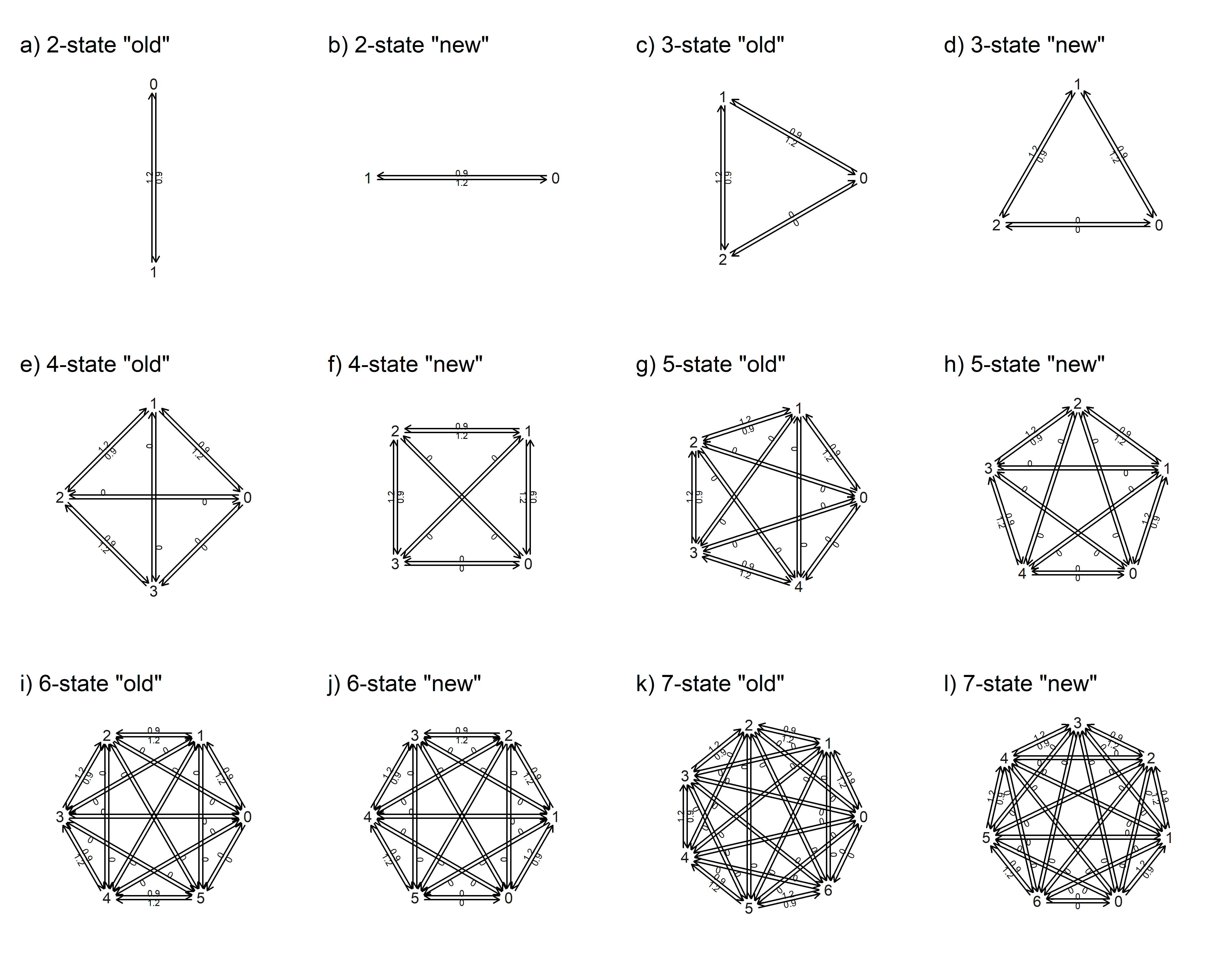Cool.

In addition, the tweet also shows a visual in which the Q matrix is graphed on top of the plotted tree.

I decided to add this functionality to `plot.Qmatrix` too, using the logical argument `add`.

In this example, I'm going to use a phylogeny & squamate digit number example subsampled from a dataset by Brandley et al. (2008).

In this case, rather than paste my fitted Q matrix right on top of the plotted tree, I'll graph my tree through only 75% of the space, and then add the fitted Mk model to the remaining quadrant.

The code below also includes a hacky method to visualize a discrete trait at the tips using thicken lines.

The arguments I used to adjust the position of the plotted Q matrix where `xlim` and `ylim`.

For instance, by setting the first value of `ylim` to be a relatively small (negative) number, and the latter value to be large, we get the Q matrix into the lower half (and so on). The matrix will always plot between -1 and 1 on both axes.

``````## read data & tree
chk<-geiger::name.check(sqTree,sqData)
sqTree.pruned<-drop.tip(sqTree,chk\$tree_not_data)
sqData.pruned<-sqData[!(rownames(sqData)%in%
chk\$data_not_tree),,drop=FALSE]
toes<-setNames(as.factor(sqData.pruned[,"rear.toes"]),
rownames(sqData.pruned))
## fit directional (loss only) trait evolution model
directional.model<-matrix(c(
0,0,0,0,0,0,
1,0,0,0,0,0,
0,2,0,0,0,0,
0,0,3,0,0,0,
0,0,0,4,0,0,
0,0,0,0,5,0),6,6,byrow=TRUE,
dimnames=list(0:5,0:5))
fitDirectional<-fitMk(sqTree.pruned,toes,model=directional.model,
pi="fitzjohn")
fitDirectional
``````
``````## Object of class "fitMk".
##
## Fitted (or set) value of Q:
##          0         1         2         3         4         5
## 0 0.000000  0.000000  0.000000  0.000000  0.000000  0.000000
## 1 0.024241 -0.024241  0.000000  0.000000  0.000000  0.000000
## 2 0.000000  0.103141 -0.103141  0.000000  0.000000  0.000000
## 3 0.000000  0.000000  0.109233 -0.109233  0.000000  0.000000
## 4 0.000000  0.000000  0.000000  0.083290 -0.083290  0.000000
## 5 0.000000  0.000000  0.000000  0.000000  0.004369 -0.004369
##
## Fitted (or set) value of pi:
## 0 1 2 3 4 5
## 0 0 0 0 0 1
## due to treating the root prior as (a) nuisance.
##
## Log-likelihood: -118.387049
##
## Optimization method used was "nlminb"
``````
``````## create plot
plotTree(sqTree.pruned,type="fan",ftype="off",
color="grey",lwd=1,part=0.75)
obj<-get("last_plot.phylo",envir=.PlotPhyloEnv)
n<-Ntip(sqTree.pruned)
cols<-setNames(grey.colors(n=length(levels(toes)),0.9,0.1),
levels(toes))
par(lend=3)
for(i in 1:Ntip(sqTree.pruned)){
cc<-if(obj\$xx[i]>0) 10 else -10
th<-atan(obj\$yy[i]/obj\$xx[i])
segments(obj\$xx[i],obj\$yy[i],
obj\$xx[i]+cc*cos(th),
obj\$yy[i]+cc*sin(th),
lwd=20,
col=cols[toes[sqTree.pruned\$tip.label[i]]])
}
legend("topleft",levels(toes),pch=15,col=cols,pt.cex=1.5,
cex=0.8,bty="n",title="number of digits")